# Helio- and Asteroseismology

INTRODUCTION  | OBSERVATIONS : the SUN  | OBSERVATIONS : STARS  | MODELS  | SOLAR OSCILLATIONS  | RESULTS
WHO ARE WE ?

## Results of solar model calculations

Model calculations follow the evolution of the Sun, from the beginning of its starting to shine due to fusion of hydrogen. The models are calculated with the known mass of the Sun : about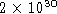kg, which we know with quite high precision from the motion of the planets around the Sun. The model of the present Sun, with an age of approximately 4.5 billion years, must also have the observed radius of about 700,000 km and total luminosity of about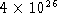Watt. Finally, the model should have the correct abundances of the chemical elements observed on the solar surface.

The results of the calculations show, among other things, how the pressure, temperature, mass density, and hydrogen content vary as a function of radius inside the Sun.

### Pressure :Here is shown the pressure variation. In this plot and the following ones the horizontal scale is the distance from the solar centre in units of the solar surface radius. Most quantities vary so much as a function of radius that it is necessary to show them on a logarithmic scale. The pressure in the solar centre is over 100 billion atmospheres, while on the visible surface it is a mere 0.1 atmospheres.

### Temperature :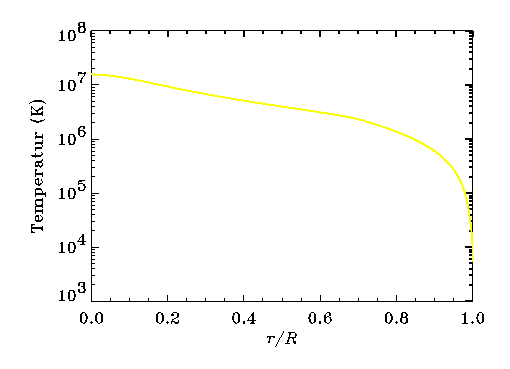The temperature at the solar centre is about 15 million Kelvin (K). It is this high temperature which causes hydrogen fusion to take place : the velocity of hydrogen nuclei is sufficiently large that they can overcome their mutual Coulomb barrier and fuse together. At the solar surface, where the light we see comes from, the temperature is nearly 6000 K.

### Density :The density at the centre of the Sun is 150 times larger than the density of water. Thus it seems rather counter-intuitive to maintain that the Sun is a ball of gas. The explanation is that the matter inside the Sun is ionized : the temperature is so high that atoms are ripped apart into free electrons and atomic nuclei. These constituents are so small that they can move about freely, as in a gas, even at a very high density. At the solar surface, the density is a thousand times smaller than the density of the atmosphere at the Earth's surface.

### Hydrogen content :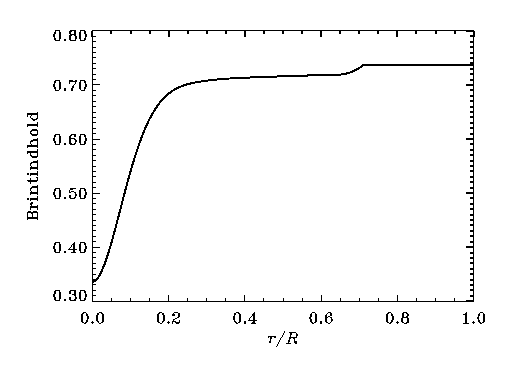The figure shows the hydrogen abundance, that is to say how many kg of hydrogen there is in one kg of matter in the Sun. When the Sun was formed the abundance was 0.71 kg of hydrogen per kg of solar matter. Of the rest 0.27 kg was helium and all other elements together massed only 0.02 kg. The hydrogen content has changed in the course of the evolution of the Sun, for two reasons :

• Hydrogen fusion has converted hydrogen into helium in the central parts, where the temperature is sufficiently high for fusion to occur. This explains the strong change of hydrogen content in the innermost roughly 20 % of the Sun. From the calculations it seems that until now about half of the hydrogen has been used up. This is consistent with the helioseismic measurements.
• Hydrogen is lighter than helium and therefore has a tendency to "float on top". This is a very slow process in the Sun, but despite that it has increased the hydrogen content in the convective layer by a few percent and caused a strong variation in its abundance just below the convective layer at r/R = 0.7.

### Sound speed :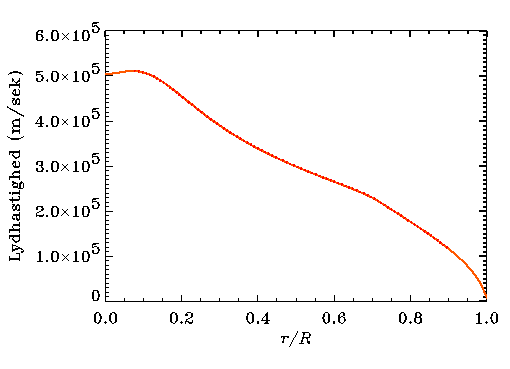The sound speed is for our purposes the most interesting model quantity because it can be compared directly with the results from observations of solar oscillations. The orange curve shows the sound speed in the model. In general it increases as one penetrates deeper into the Sun, with the increasing temperature. However in the central parts there is a small decrease in the sound speed, because of the decrease in hydrogen abundance and the increase in helium. The dotted curve shows the sound speed obtained from analysis of the oscillation frequencies. It appears that the model isn't half bad !